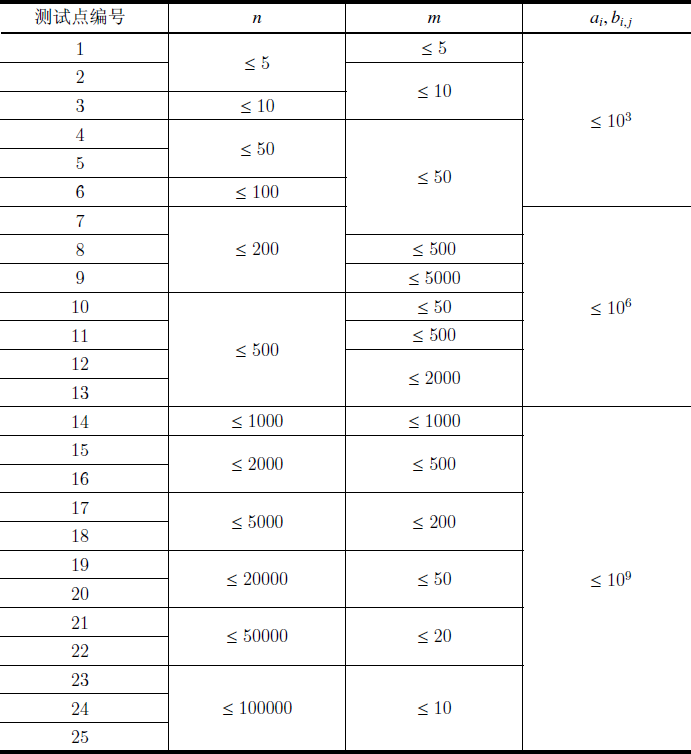…

# YZOJ P2358 [ZJOI 2012]Naive – Matrix

• ### 数据规模与约定

Source: BZOJ 2658…

# [校内训练20161216] T2 版本管理git

• ### 题目描述

$$F$$ 公司有一个项目 $$U$$，这个项目由一个字符串构成。版本 $$0$$ 为空串。有 $$n$$ 个开发者按顺序对这个项目进行了修改，其中第 $$i$$ 个开发者将第 $$p_i ( 0 \le p_i < i)$$ 个版本的开头添加上一个字符 $$c_i (1 \le c_i \le m)$$ 作为新的版本 $$i$$。

…

# YZOJ P3361 [校内训练20171117]数点问题

• ### 题目描述

$$k$$ 维空间内有两个点集 $$A=\{X_1,X_2,\ldots,X_m\}$$，$$B=\{Y_1,Y_2,\ldots,Y_n\}$$，每个点的坐标是一个 $$k$$ 元组 $$(x_1,x_2,\ldots,x_k)$$。我们称点 $$X(x_1,x_2,\ldots,x_k)$$ 控制点 $$Y(y_1,y_2,\ldots,y_k)$$ 当且仅当 $$\forall 1\le i\le k,x_i>y_i$$，记为 $$X>Y$$。数点问题是要求计算点 $$X_i$$ 能控制 $$B$$ 中的点数 $$c_i$$，即 $$c_i=\left| \{Y \in B\ |\ X_i > Y\} \right|$$。

# YZOJ P3791 餐馆

• ### 数据规模与约定Source：ARC067 F – Yakiniku

# [FJWC2019 Day1] 全连

• ### 样例说明

$$S=\{1,3,5\}$$，答案 $$=2 \times 3 + 2 \times 2 + 2 \times 4 = 18$$ 。

• ### 数据规模与约定

YZOJ P4257, YZOJ P3993…

# YZOJ P3314 计算器

• ### 题目描述

< 表达式 > ：  < 运算数 1>< 运算符 1>< 运算数 2>< 运算符 2> $$\cdots$$ < 运算符 $$k-1$$>< 运算数 $$k$$>（ $$k$$ 为正整数）

• ### 数据规模与约定

$$1 \leq \left| s \right| \leq 10^4$$

…